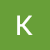# What is Machine Learning?

Machine learning focuses on applications that learn from experience and improve their decision-making or predictive accuracy over time.

In this article I will explain what is Machine Learning?, where it is used, types of machine learning and much more.

Machine Learning is an application of artificial intelligence that provides system ability to learn and improve from experience without being explicitly programmed. Machine learning is used to develop computer programs that can access data and use the data to learn for themselves.

Machine-learning algorithms use statistics to find patterns in massive amounts of data. A Machine Learning system learns from historical…

# Finds Algorithm in Machine Learning

Hi guys, in this article I will implement and demonstrate the FIND-S algorithm for finding the most specific hypothesis based on a given set of training data samples.

In finds algorithm , we initialize hypothesis as an array of phi, thein in the first step we replace it with the first positive row of our dataset which is most specific hypothesis.

In next step, we will traverse the dataset and check if the target value of dataset is positive or not, we will only consider positive value. if the value is positive we will traverse that row from start to…

# Linear Regression- Machine Learning

Get a complete code of linear regression algorithm from scratch at ml-lab

Linear regression is one of the easiest and most popular Machine Learning algorithms. It is a statistical method that is used for predictive analysis. Linear regression makes predictions for continuous/real or numeric variables such as sales, salary, age, product price, etc.

Linear regression algorithms show a linear relationship between a dependent (y) and one or more independent (y) variables, hence called linear regression. …

# Linux Directories Explained

/root (/): /root is the home directory of the superuser (also known as the “Administrator”) of the system. All the directories in the Linux system come under the root directory which is represented by a forward slash (/). Everything in your system can be found under this root directory even if they are stored in different virtual or physical devices.

# How install Virtual Machine on Windows.

In this article I am going to explain how to install a virtual machine on windows operation system.

Installing VirtualBox :